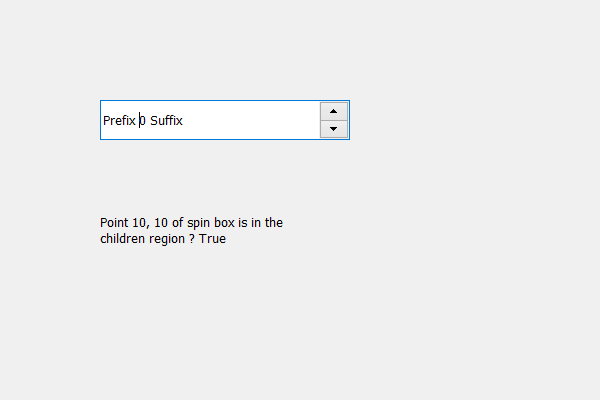Related Articles
PyQt5 QSpinBox – Checking if certain point is in the Children Region
• Last Updated : 19 May, 2020

In this article we will see how we can check the certain point lies in the children region of spin box, children region holds the combined region occupied by the spin box’s children. In order to do this we use `childrenRegion` method.

Note : The point should be in the Spin box co-ordinates system

In order to do this we use contains method with the children region object of the spin box

Syntax : children_region.contains(point)

Argument : It takes QPoint object as argument

Return : It returns bool object

Below is the implementation

 `# Write Python3 code here# importing libraries ` `from` `PyQt5.QtWidgets ``import` `*`  `from` `PyQt5 ``import` `QtCore, QtGui ` `from` `PyQt5.QtGui ``import` `*`  `from` `PyQt5.QtCore ``import` `*`  `import` `sys ` ` `  ` `  `class` `Window(QMainWindow): ` ` `  `    ``def` `__init__(``self``): ` `        ``super``().__init__() ` ` `  `        ``# setting title ` `        ``self``.setWindowTitle(``"Python "``) ` ` `  `        ``# setting geometry ` `        ``self``.setGeometry(``100``, ``100``, ``600``, ``400``) ` ` `  `        ``# calling method ` `        ``self``.UiComponents() ` ` `  `        ``# showing all the widgets ` `        ``self``.show() ` ` `  `        ``# method for widgets ` `    ``def` `UiComponents(``self``): ` `        ``# creating spin box ` `        ``self``.spin ``=` `QSpinBox(``self``) ` ` `  `        ``# setting geometry to spin box ` `        ``self``.spin.setGeometry(``100``, ``100``, ``250``, ``40``) ` ` `  `        ``# setting range to the spin box ` `        ``self``.spin.setRange(``0``, ``999999``) ` ` `  `        ``# setting prefix to spin ` `        ``self``.spin.setPrefix(``"Prefix "``) ` ` `  `        ``# setting suffix to spin ` `        ``self``.spin.setSuffix(``" Suffix"``) ` ` `  `        ``# getting the children region ` `        ``children_region ``=` `self``.spin.childrenRegion() ` ` `  `        ``# creating a label ` `        ``label ``=` `QLabel(``self``) ` `        ``label.setWordWrap(``True``) ` ` `  `        ``# setting geometry to the label ` `        ``label.setGeometry(``100``, ``200``, ``200``, ``60``) ` ` `  `        ``# getting bounding rectangle from the children region ` `        ``bounding_rectangle ``=` `children_region.contains(QPoint(``10``, ``10``)) ` ` `  `        ``# setting text to the label ` `        ``label.setText(``"Point 10, 10 of spin box is in the children region "` `                      ``"? "` `+` `str``(bounding_rectangle)) ` ` `  ` `  ` `  `# create pyqt5 app ` `App ``=` `QApplication(sys.argv) ` ` `  `# create the instance of our Window ` `window ``=` `Window() ` `window.spin.setFocus() ` `# start the app ` `sys.exit(App.``exec``()) `

Output :Attention geek! Strengthen your foundations with the Python Programming Foundation Course and learn the basics.

To begin with, your interview preparations Enhance your Data Structures concepts with the Python DS Course.

My Personal Notes arrow_drop_up
Recommended Articles
Page :